Yesterday, I came across a nice article untitled “Here’s Waldo: Computing the optimal search strategy for finding Waldo“, written by Randal S. Olson. In this article, Randal explains that he has devoted some time to try to compute the optimal search strategy for finding Waldo. To that end, he has used some machine learning techniques.
From an image provided by Slate (Here’s Waldo, 2013, by Ben Blatt), Randal S. Olson retrieved the coordinates of 68 different locations of Waldo, and kindly shared the data afterwards.

As a first step, Randal has estimated a kernel density of the points. Here is what he finds:Kernel Density estimate of Waldos location (Randal S. Olson)

I decided to spent a few minutes reproducing this, but applying some correction to the estimation regarding the border effects. Some time Ago, Arthur Charpentier (aka @freakonometrics) and I worked on kernel density estimation of points. We provided a simple way of correcting a bias effect near frontier, inspired by Ripley’s circumference method (see the article in Geoinformatica, or some notes on my blog, or on Arthur’s). The problem with the estimation of the density of the locations of Waldo is that those locations are compelled to lie on the double page. Hence, we now for sure that Waldo will not be found outside the rectangle that corresponds to the double page. So, when we estimate the kernel density of the points, we face a bias near the borders, as we expect to find some points outside the rectangle.

I used the codes we shared with Arthur on the kernel density estimation to estimate the kernel density estimation of Waldo’s location.

After loading the data in R, I plotted the points. This is what I got: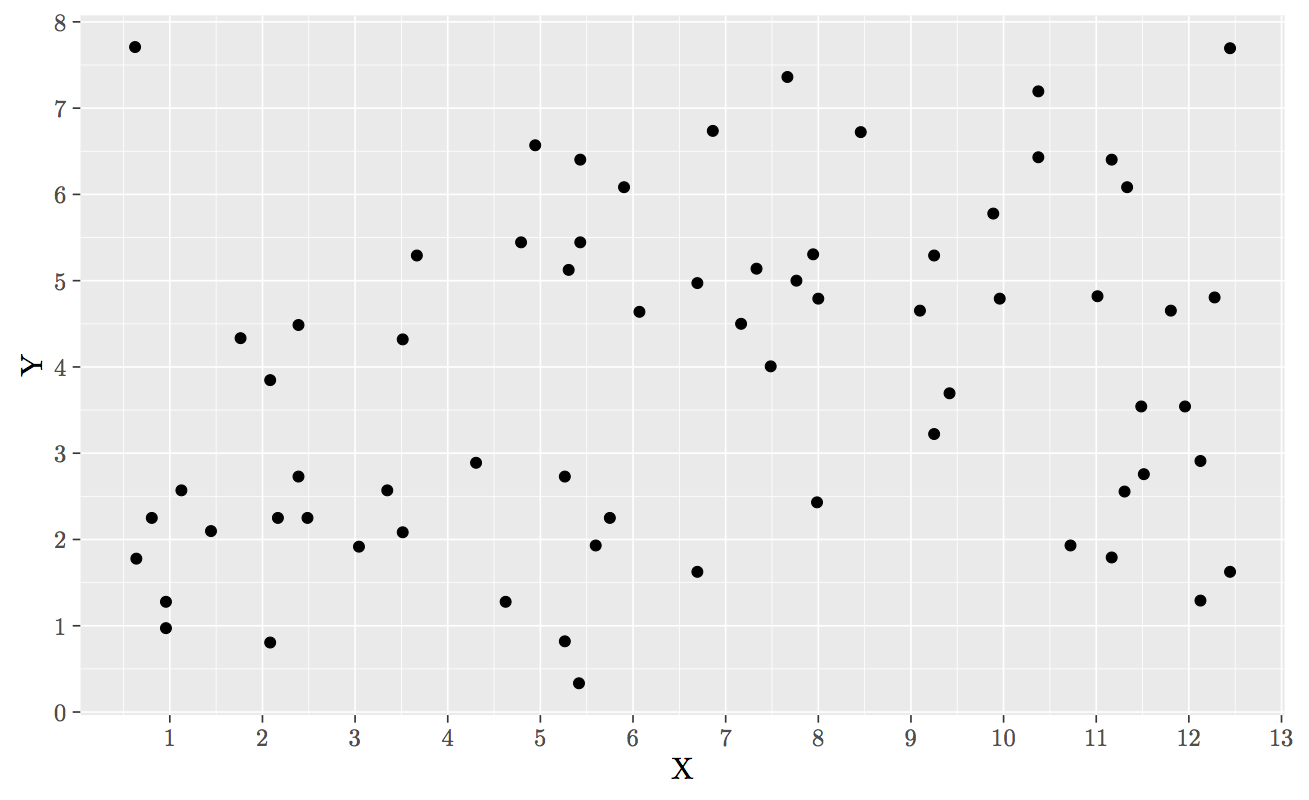Location of Waldo

I guess that the rectangle on which we have to search for Waldo is a 13 times 8 rectangle.


bounding_polygon <- data.frame(x = c(0, 0, 13, 13, 0), y = c(0, 8, 8, 0, 0))
bounding_polygon_gpc <- as(bounding_polygon, "gpc.poly")


I run the estimation:


# Estimation with border correction
smoothed_kde <- sKDE(U = waldo[, c("X", "Y")],
polygon = bounding_polygon_gpc,
optimal=TRUE, parallel = FALSE)

# Estimation without border correction
smoothed_kde_nc <- sKDE_without_c(U = waldo[, c("X", "Y")],
polygon = bounding_polygon_gpc,
optimal=TRUE)


Then I plot the results on two different graphs. The first one shows the estimation without correcting for the bias effect near borders, and the second one shows the estimation with the correction.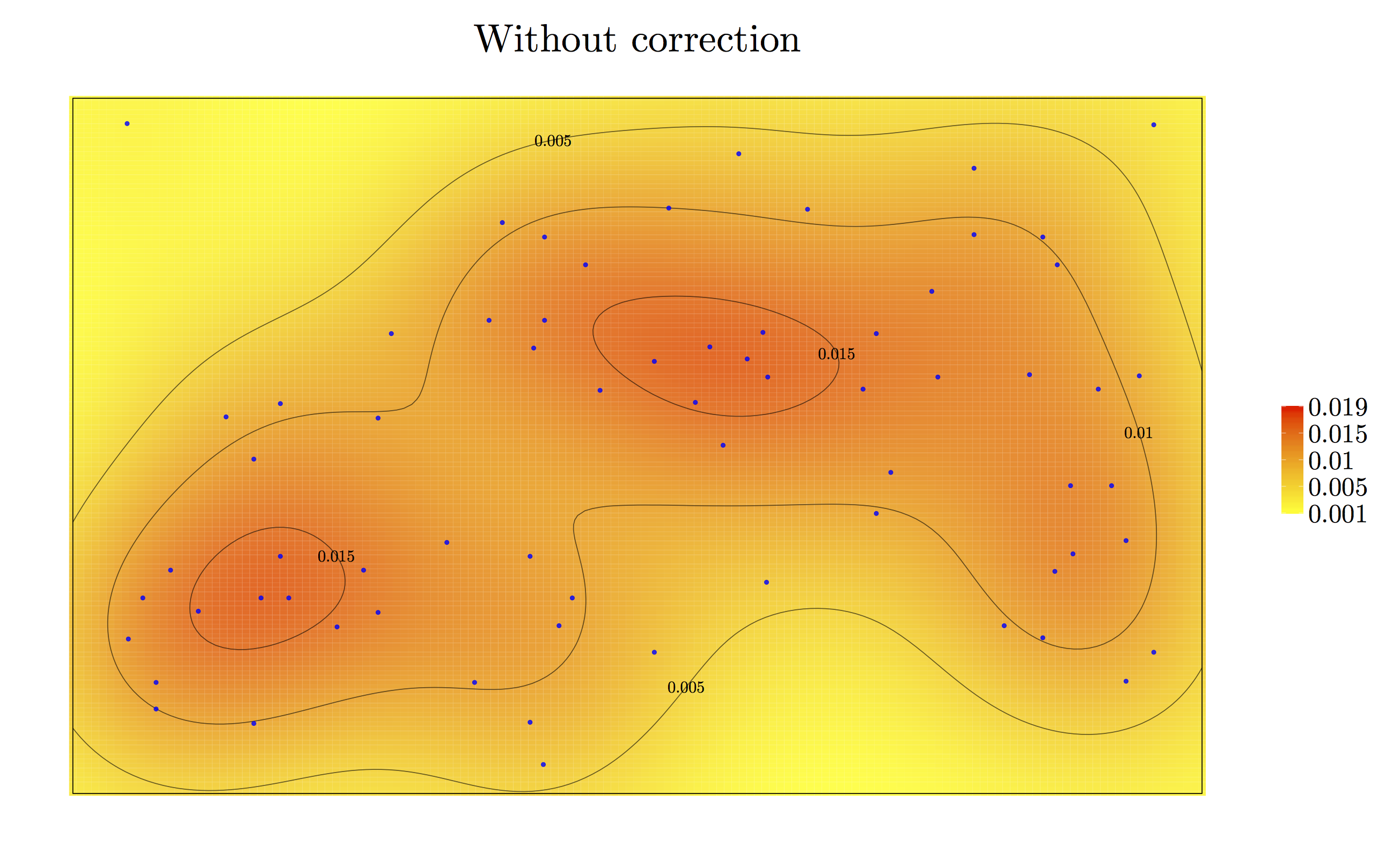Kernel density estimation of Waldo’s location, without correction of the border bias effect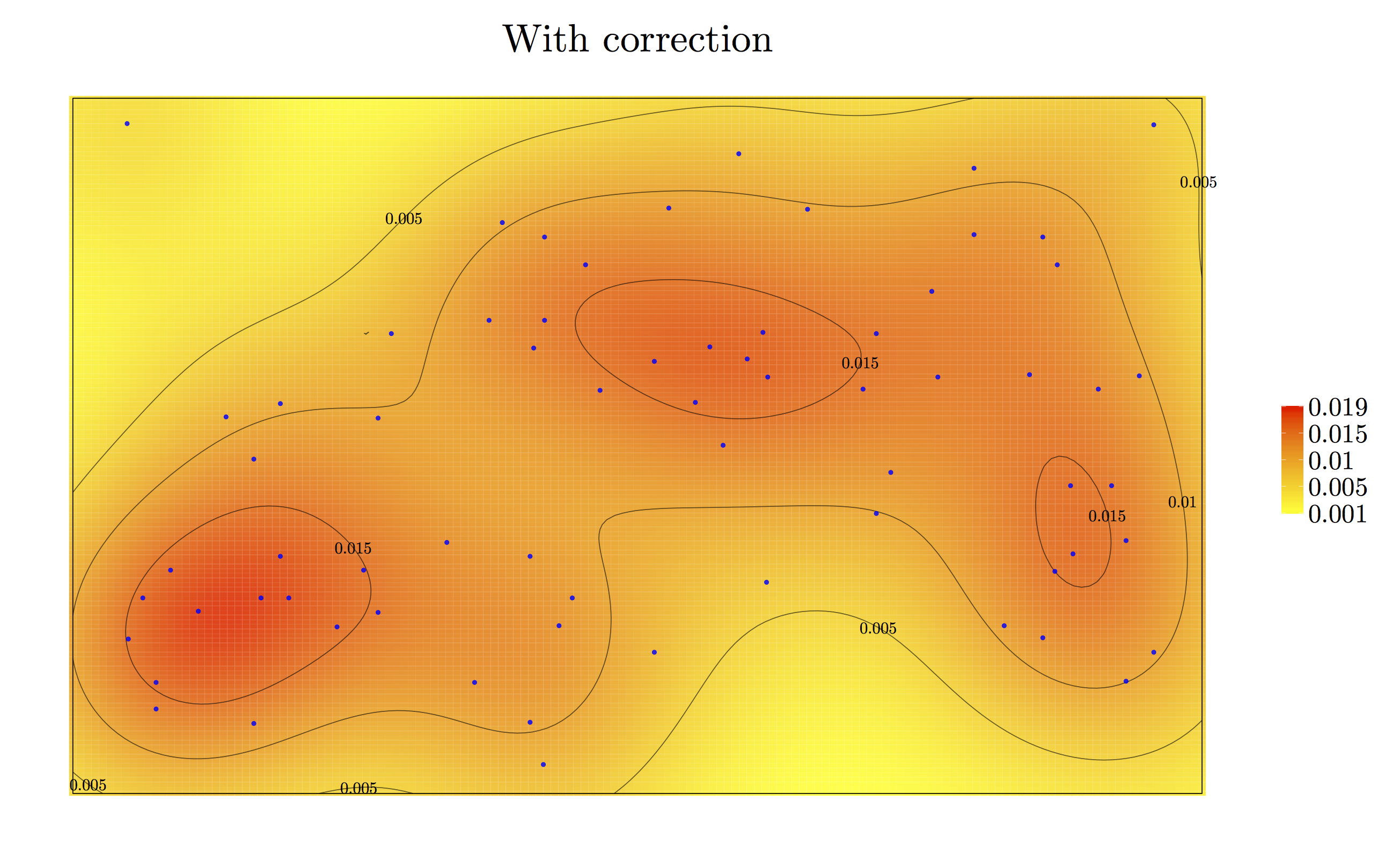Kernel density estimation of Waldo’s location, with correction of the border bias effect

As we can see, the estimation of the density becomes higher on the right border of the double page.

However, as noted by Randal, there is always a postcard on the top-left corner of the page, where the reader can find some descriptions. So, behind this postcard, there is absolutely no chance of finding Waldo. Hence, I decided to roughly position the postcard and then to remove it from the bounding rectangle where Waldo is to be found.


postcard <- data.frame(x = c(1, 1, 3, 3, 1), y = c(5, 7.5, 7.5, 5, 5))
postcard_gpc <- as(postcard, "gpc.poly")


I remove this area from the rectangle:


bound_pol <- gpclib::setdiff(bounding_polygon_gpc, postcard_gpc)


And I estimate the density again:


# Estimation with correction of the border bias
smoothed_kde_post <-
sKDE(U = waldo[, c("X", "Y")],
polygon = bound_pol,
optimal=TRUE, parallel = FALSE)

# Estimation without the correction
smoothed_kde_post_nc <-
sKDE_without_c(U = waldo[, c("X", "Y")],
polygon = bound_pol,
optimal=TRUE)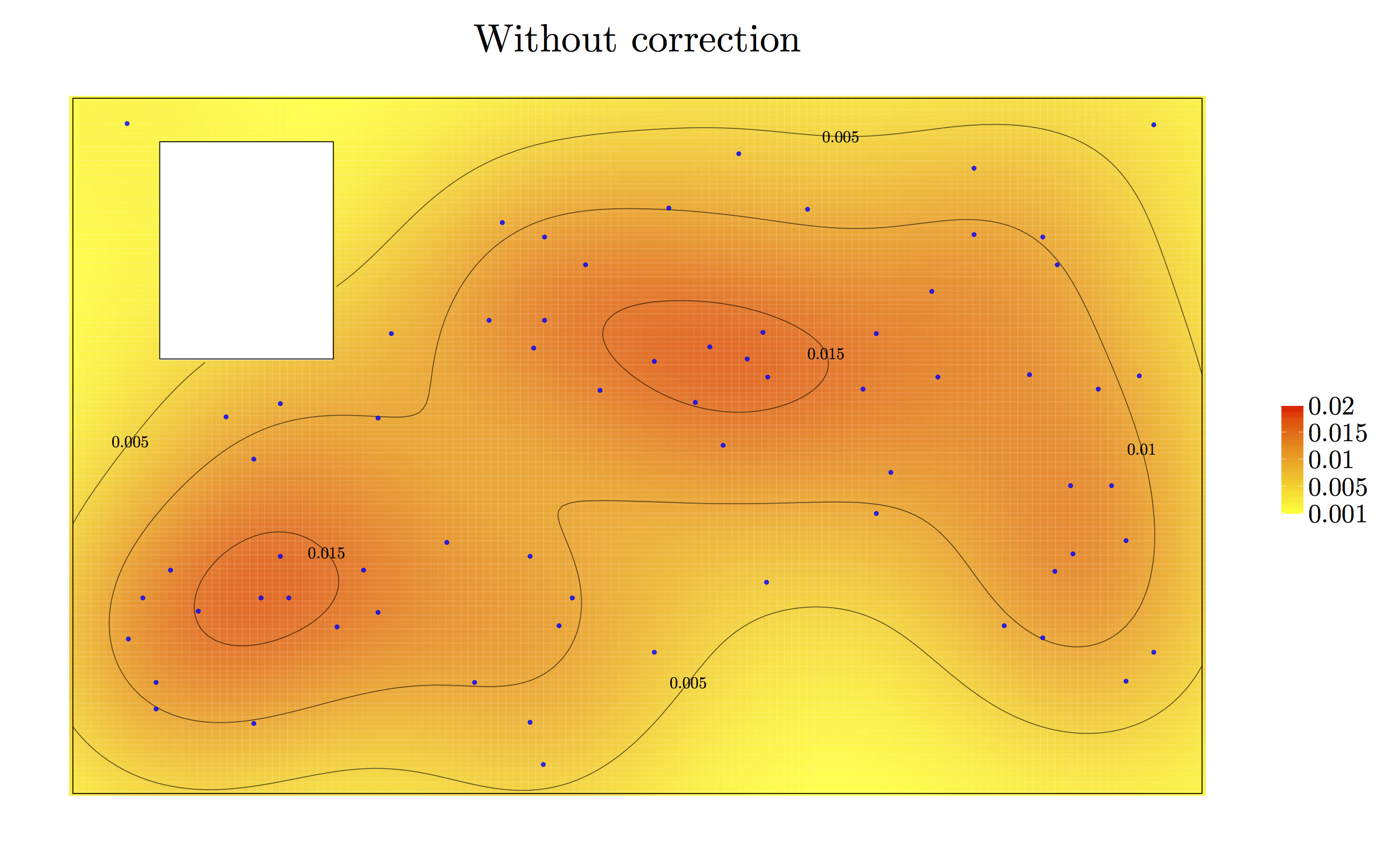Kernel density estimation of Waldo’s location, without correction of the border bias effect, removing the postcard on the left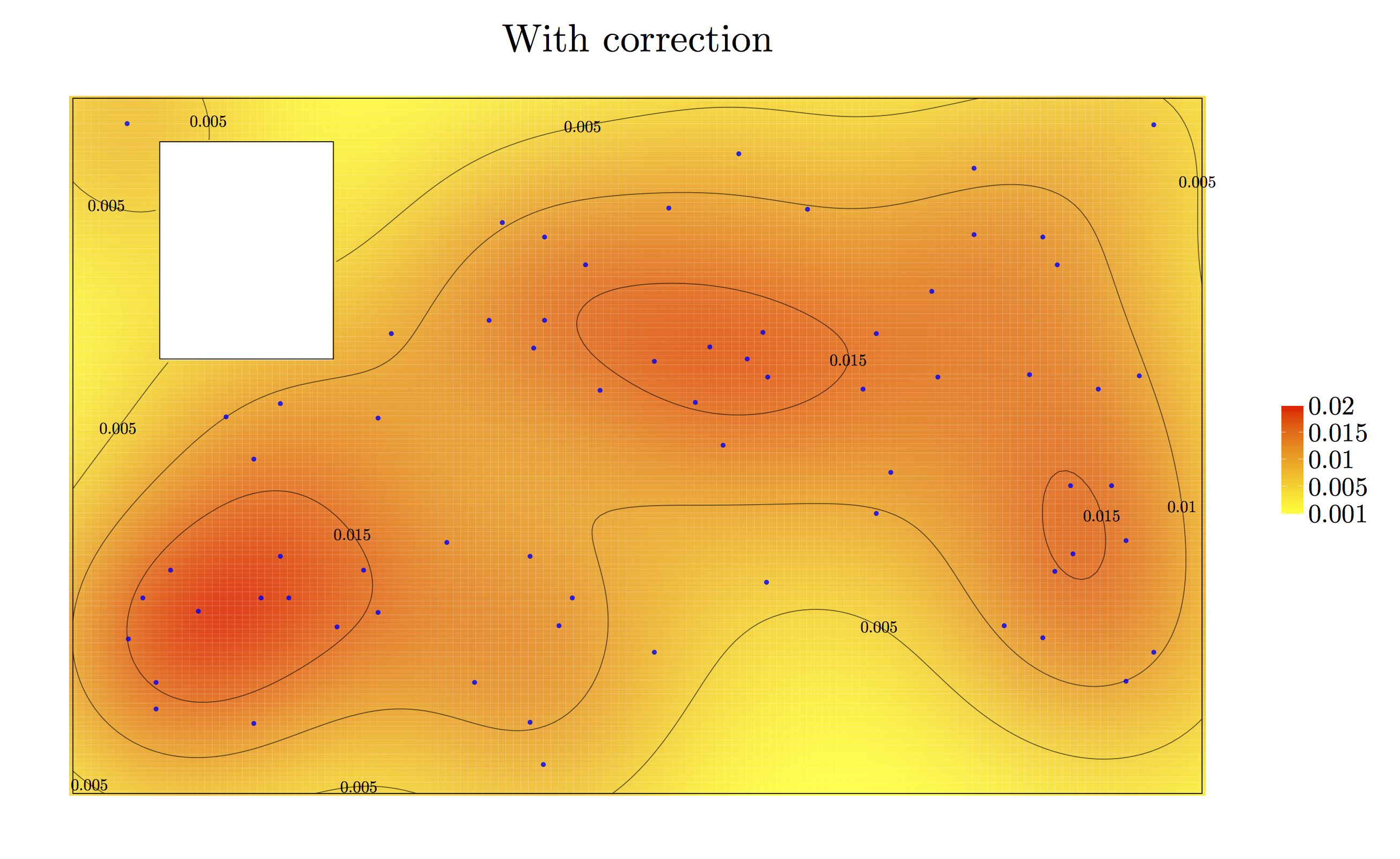Kernel density estimation of Waldo’s location, with correction of the border bias effect, removing the postcard on the left

Just for fun, here is a gif showing the estimation without correction (and without caring about the postcard), and with correction (and using the extra information about the existence of the postcard):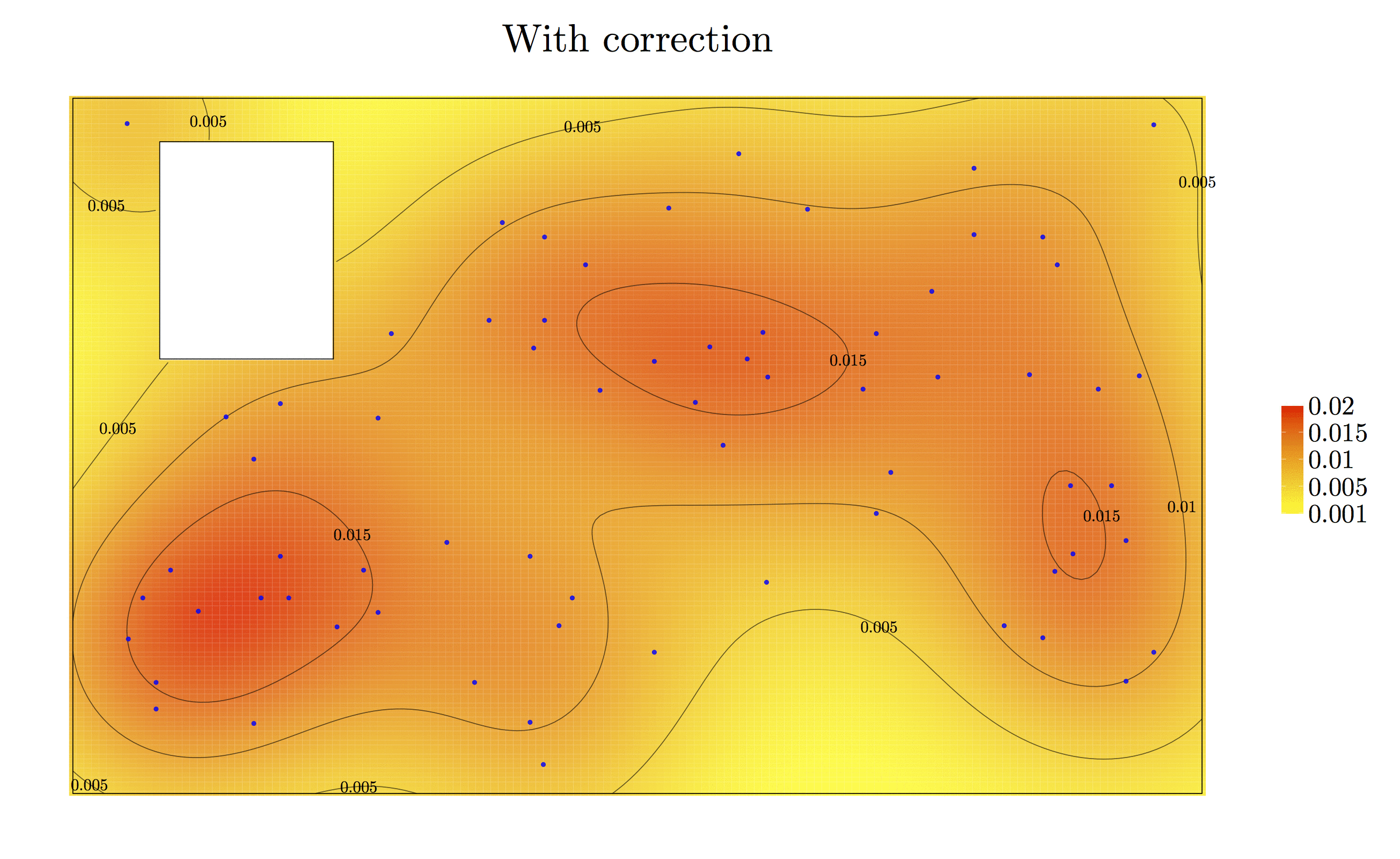The complete R codes are available here.